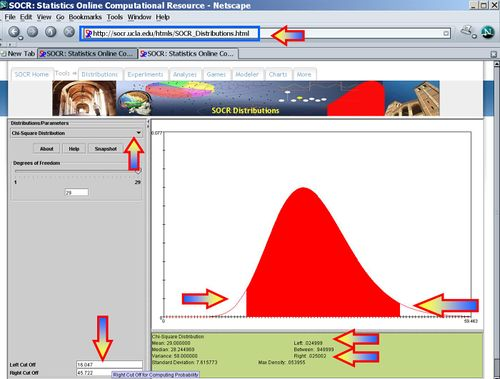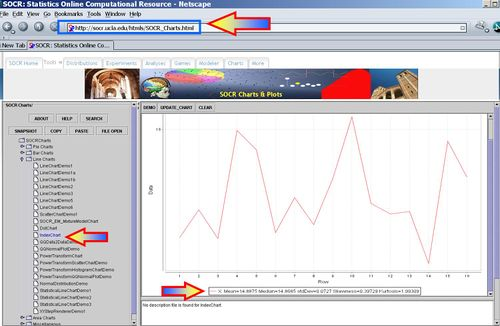# Difference between revisions of "AP Statistics Curriculum 2007 Hypothesis Var"

## General Advance-Placement (AP) Statistics Curriculum - Testing a Claim about a Standard Deviation or Variance

Assessing the amount of variation in a process, natural phenomenon or an experiment is of paramount importance in many fields. For instance, a computer manufacturer may dismiss a batch of computer chips if they vary more than certain tolerance levels in their clock-speed, heat emissions or energy consumptions.

### Background

Recall that the sample-variance (s2) is an unbiased point estimate for the population variance $$\sigma^2$$, and similarly, the sample-standard-deviation (s) is a good point estimate for the population-standard-deviation $$\sigma$$.

The sample-variance is roughly Chi-square distributed: $\chi_o^2 = {(n-1)s^2 \over \sigma^2} \sim \Chi_{(df=n-1)}^2$

### Testing a Claim about the Variance ($$\sigma^2$$)

For Normally distributed random variables, given $$H_o: \sigma^2 = \sigma_o^2$$ vs. $$H_1: \sigma^2 \not= \sigma_o^2$$ , then $${(n-1) s^2 \over \sigma_o^2}$$ has a $$\Chi^2_{(df=n - 1)}$$ distribution, where $$s^2$$ is the sample variance.

Notice that the Chi-square distribution is not symmetric (it is positively skewed) and therefore, there are two critical values for each level of confidence $$(1-\alpha)$$. The value $$\chi_L^2$$ represents the left-tail critical value and $$\chi_R^2$$ represents the right-tail critical value. For various degrees of freedom and areas, you can compute all critical values either using the SOCR Chi-Square Distribution or using the SOCR Chi-square distribution calculator.

• Example: A random sample of size 30 drawn from a Normal distribution has sample-variance $$s^2 = 5$$. Test at the $$\alpha=0.05$$ level of significance if this is consistent with $$H_o: \sigma^2 = 2$$.
Test statistic$\chi_o^2 = {29\times 5 \over 2} = 72.5$.
Left and Right Chi-Square critical values (for $$\alpha = 0.05$$) are $$\chi_L^2=16.047$$ and $$\chi_R^2=45.722$$. Since, $$\chi_o^2 = 72.5 > \chi_R^2=45.722$$, $$H_o$$ is rejected at the $$\alpha=0.05$$ level of significance.### Testing a Claim about the Standard Deviation ($$\sigma$$)

As the standard deviation is just the square root of the variance ($$\sigma = |\sqrt{\sigma^2}|$$), we do significance testing for the standard deviation analogously.

For Normally distributed random variables, given $$H_o: \sigma = \sigma_o$$ vs. $$H_1: \sigma \not= \sigma_o$$ , then $${(n-1) s^2 \over \sigma_o^2}$$ has a $$\Chi^2_{(df=n - 1)}$$ distribution, where $$s^2$$ is the square of the sample standard deviation.

### Hands-on Activities

• Formulate appropriate hypotheses and assess the significance of the evidence to reject the null hypothesis for the population standard deviation ($$\sigma$$) assuming the observations below represent a random sample from the liquid content (in fluid ounces) of 16 beverage cans and can be considered as Normally distributed. Use a 90% level of confidence ($$\alpha=0.1$$).
 14.816 14.863 14.814 14.998 14.965 14.824 14.884 14.838 14.916 15.021 14.874 14.856 14.86 14.772 14.98 14.919
• Hypotheses$H_o: \sigma = 0.06 (\sigma_o)$ vs. $$H_1: \sigma \not= 0.06$$ .
• Get the sample statistics from SOCR Charts (e.g., Index Plot); Sample-Mean=14.8875; Sample-SD=0.072700298, Sample-Var=0.005285333.• Identify the degrees of freedom ($$df=n-1=15$$).
• Test Statistics$\Chi_o^2 = {(n-1)s^2 \over \sigma_o^2} \sim \Chi_{(df=n-1)}^2.$
• Significance Inference$\chi_o^2 = {15\times 0.005285333 \over 0.06^2}=22.022221$
P-value=$$P(\Chi_{(df=n-1)}^2 > \chi_o^2) = 0.107223$$. This p-value does not indicate strong evidence in the data to reject a possible population standard deviation of 0.06.
Error creating thumbnail: File missing

### More examples

• You randomly select and measure the contents of 15 bottles of cough syrup. The results (in fluid ounces) are shown. Formulate and test hypotheses about the standard deviation ($$\sigma_o=0.025$$) assuming the contents of these cough syrup bottles are Normally distributed. Is there data-driven evidence suggesting that the standard-deviation of the fluids in the bottles is not at an acceptable level?
 4.211 4.246 4.269 4.241 4.26 4.293 4.189 4.248 4.22 4.239 4.253 4.209 4.3 4.256 4.29
• The gray whale has the longest annual migration distance of any mammal. Gray whales leave Baja, California, and western Mexico in the spring, migrating to the Bering and Chukchi seas for the summer months. Tracking a sample of 50 whales for a year provided a sample mean migration distance of 11,064 miles with a standard deviation of 860 miles. Assume that the population of migration distances is Normally distributed to formulate and test hypotheses for the population standard deviation ($$\sigma_o=500$$).
• Use the hot-dogs dataset to formulate and test hypotheses about the population standard deviation of the sodium content in the poultry hot-dogs ($$\sigma_o=70$$).